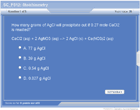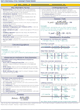How to Learn in 24 Hours?

 Need Help? M-F: 9am-5pm(PST): Toll-Free: (877) RAPID-10 or 1-877-727-4310 24/7 Online Technical Support: The Rapid Support Center vip@rapidlearningcenter.com Secure Online Order:Need Proof? Testimonials by Our Users

 Rapid Learning Courses: MCAT in 24 Hours (2021-22) USMLE in 24 Hours (Boards) Chemistry in 24 Hours Biology in 24 Hours Physics in 24 Hours Mathematics in 24 Hours Psychology in 24 Hours SAT in 24 Hours ACT in 24 Hours AP in 24 Hours CLEP in 24 Hours DAT in 24 Hours (Dental) OAT in 24 Hours (Optometry) PCAT in 24 Hours (Pharmacy) Nursing Entrance Exams Certification in 24 Hours eBook - Survival Kits Audiobooks (MP3)

 Tell-A-Friend: Have friends taking science and math courses too? Tell them about our rapid learning system.Home »  Chemistry »  SAT Chemistry

The Math of Chemical Reactions-Stoichiometry

 Topic Review on "Title": Stoichiometry is the culmination of many concepts in chemistry.  It integrates dimensional analysis, moles and molar mass, molar volumes of gases, concentrations and balanced chemical equations to use information about one species in a reaction to determine information about another species in the same reaction. Review Chemical equations are used to relate reactants and products in a chemical reaction.  Dimensional analysis is a technique that uses ratios of equivalents to convert units.  The KUDOS method is a method for solving word problems.  Molarity is a concentration unit showing the ratio of moles of solute to volume of solution. Mole-Mole problems The balanced equation gives the ratio of moles of each species in the reaction.  The balanced equation is used to determine mole ratios for use in dimensional analysis when converting from the moles of one species to the moles of another.  Moles is the only unit that may be used to convert from one chemical to another—mass or volume cannot be used to convert between chemicals, just within the same one. Mole-Mass & Mass-Mass problems Molar mass is an equivalent between the mass in grams and moles of a substance.  The molar mass is used to convert between moles of a substance and mass in a stoichiometry problem. Mole-Volume problems for solutions The concentration, in molarity, of a solution gives the equivalent for moles of the solute and liters of solution.  The molarity can be used to convert between moles and volume of a solution in stoichiometry problems. Mole-Volume problems for gases The volume of 1 mole of any gas at standard temperature and pressure is 22.4 L.  The molar volume of a gas can be used to convert between moles and volume of a gas at STP in stoichiometry problems.  If a problem asks for the volume at non-standard temperature or pressure, find the STP volume using stoichiometry and then use the appropriate gas law to convert to the desired temperature or pressure.

Rapid Study Kit for "Title":
 Flash Movie Flash Game Flash Card Core Concept Tutorial Problem Solving Drill Review Cheat Sheet"Title" Tutorial Summary : Stoichiometry uses the principles of dimensional analysis to use information about one species in a chemical reaction to determine information about a different species in the same reaction.  This tutorial guides you through stoichiometric calculations involving moles, mass, solution volume and gas volume.

 Tutorial Features: Specific Tutorial Features: Use of real-life analogies to explain stoichiometric concepts Series Features: Concept map showing inter-connections of new concepts in this tutorial and those previously introduced. Definition slides introduce terms as they are needed. Visual representation of concepts Animated examples—worked out step by step A concise summary is given at the conclusion of the tutorial.

 "Title" Topic List: Review Chemical equations Dimensional Analysis KUDOS method Molarity Stoichiometry Mole-Mole problems Mole-mass problems Mass-mass problems Mole-volume problems for solutions Mole-volume problems for gases

See all 24 lessons in college chemistry, including concept tutorials, problem drills and cheat sheets:
Teach Yourself SAT Chemistry Visually in 24 Hours